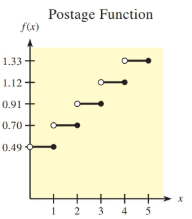Chapter 9.2, Problem 52E### Mathematical Applications for the ...

11th Edition
Ronald J. Harshbarger + 1 other
ISBN: 9781305108042

#### Solutions

Chapter
Section### Mathematical Applications for the ...

11th Edition
Ronald J. Harshbarger + 1 other
ISBN: 9781305108042
Textbook Problem

# Postage costs First-class postage for a standard letter is 49 cents for the First ounce or part of an ounce that a letter weighs plus an additional 21 cents for each additional ounce or part of an ounce. Use the table or graph of the postage function, f(x), to determine the following.(a) lim x → 2.5 f ( x ) (b) f ( 2.5 ) (c) Is f ( x ) continuous at 2.5?(d) lim x → 4 f ( x ) (e) f ( 4 ) (f) Is f ( x ) continuous at 4? Weight x Postage f(x) 0 < x ≤ 1 \$0.49 1 < x ≤ 2 0.70 2 < x ≤ 3 0.91 3 < x ≤ 4 1.12 4 < x ≤ 5 1.33(a)

To determine

The limit of the function limx2.5f(x).

Explanation

Given information:

First class postage for a standard letter is 49 cents for the first ounce or part of an ounce that a letter weighs plus an additional 21 cents for each additional ounce or part of an ounce.

Calculation:

From the graph the value of f(x)=0

(b)

To determine

The value of the function f(2.5).

(c)

To determine

Whether f(x) is continuous at 2.5.

(d)

To determine

The limit of the function limx4f(x).

(e)

To determine

The value of the function f(4).

(e)

To determine

Whether f(x) is continuous at 4.

### Still sussing out bartleby?

Check out a sample textbook solution.

See a sample solution

#### The Solution to Your Study Problems

Bartleby provides explanations to thousands of textbook problems written by our experts, many with advanced degrees!

Get Started

#### Explain what happens during each of the two stages of the two-factor ANOVA.

Essentials of Statistics for The Behavioral Sciences (MindTap Course List)

#### Find g(1)+g(2)+g(3)+....+g(50), given that g(x)=124x.

Finite Mathematics for the Managerial, Life, and Social Sciences

#### The scalar projection of on is:

Study Guide for Stewart's Multivariable Calculus, 8th